# Civil Engineering - Surveying

### Exercise :: Surveying - Section 2

26.

The operation of making the algebraic sum of latitudes and departures of a closed traverse, each equal to zero, is known

 A. balancing the sights B. balancing the departures C. balancing the latitudes D. balancing the traverse.

Explanation:

No answer description available for this question. Let us discuss.

27.

If the sight distance (S) is equal to the length of the vertical-curve (2l) joining two grades g1% and - g2%, the height of the apex will be

 A.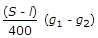B.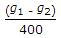C.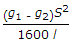D. none of these.

Explanation:

No answer description available for this question. Let us discuss.

28.

Orientation of a plane table by solving two point problem is only adopted when

 A. saving of time is a main factor B. better accuracy is a main factor C. given points are inaccessible D. none of these.

Explanation:

No answer description available for this question. Let us discuss.

29.

A back sight

 A. is always taken on a point of known elevation or can be computed B. is added to the known level to obtain the instrument height C. taken on an inverted staff is treated as negative D. all the above.

Explanation:

No answer description available for this question. Let us discuss.

30.

If the area calculated form the plan plotted with measurements by an erroneous chain, accurate area of the plan is

 A.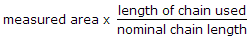B.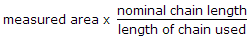C.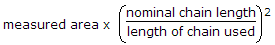D.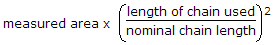E. none of the above